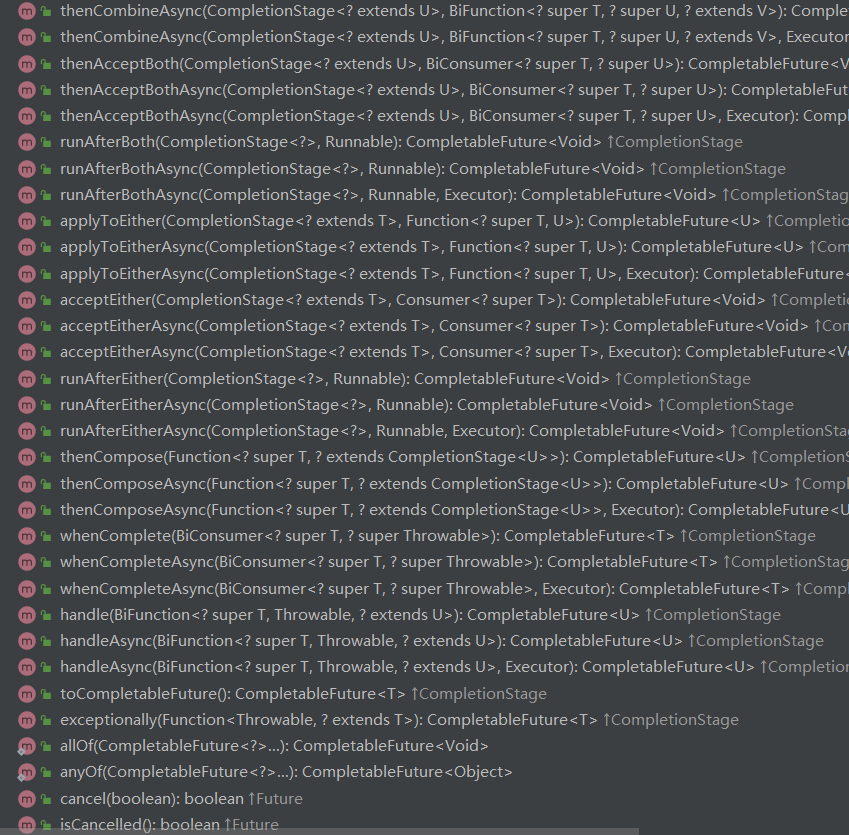函数式编程杂谈

2019/09/16 11:47

一、编程范式综述

1.命令式

1. 运算语句将结果存入存储器中以便日后使用；

2. 循环语句使得一些语句可以被反复运行；

3. 条件分支语句允许仅当某些条件成立时才运行某个指令集合；

4. 以及存有争议的类似goto这样的无条件分支语句。

3.函数式

1. 减少了可变量的声明，程序更为安全；

2. 相比命令式编程，少了非常多的状态变量的声明与维护，天然适合高并发多线程并行计算等任务，我想这也是函数是编程近年又大热的重要原因之一；

3. 代码更为简洁，但是可读性是高是低也依赖于不同场景、仁者见仁智者见智。

二、函数式编程的一些趣味用法

1.Closure(闭包)

public class OutClass {

private void helloWorld() {
System.out.println("Hello World!");
}

public InnerClass getInnerClass() {
return new InnerClass();
}

public class InnerClass {
public void hello() {
helloWorld();
}
}

/**
* @param args
*/
public static void main(String[] args) {
// 在外部使用OutClass的private方法
new OutClass().getInnerClass().hello();
}
}

public class C implements A {

@Override
public void m() {
//...
}

private void o() {
//...
}

public D getD() {
return new D();
}

class D implements B {
@Override
public void m() {
o();
}
}

public static void main(String[] args) {
C c = new C();
c.m();
c.getD().m();
}
}

2.Currying(柯里化)

var calculator = function(x, y, z){
return(x + y)* z;
}

var calculator=function(x){
return function(y){
return function(z){
return(x + y)* z;
};
};
};

Java 中的柯里化实现

Function<Integer, Function<Integer, Function<Integer, Integer>>> currying =
new Function<Integer, Function<Integer, Function<Integer, Integer>>>() {

@Override
public Function<Integer, Function<Integer, Integer>> apply(Integer x) {
return new Function<Integer, Function<Integer, Integer>>() {

@Override
public Function<Integer, Integer> apply(Integer y) {

return new Function<Integer, Integer>() {
@Override
public Integer apply(Integer z) {
return (x + y) * z;
}
};
}
};
}
};

//在这里，我们可以发现，虽然依次输入2、7，但是我们并不会计算结果，而是等到最后输入结束时才会返回值。
Function function1 = curryingFun().apply(2);//返回的是函数
Function function2 = curryingFun().apply(2).apply(7);//返回的是函数
Integer value = curryingFun().apply(2).apply(7).apply(3);//参数全部输入，返回最后的值

（1）支持的观点

• 延迟计算，只有在最后的输入结束才会进行计算;

• 当你发现你要调用一个函数，并且调用参数都是一样的情况下，这个参数就可以被柯里化，以便更好的完成任务;

• 优雅的写法，语义更有表达力;

（2）不过也有一些人持反对观点，参数的不确定性、排查错误困难。

3.Promise

Promise 是异步编程的一种解决方案，比传统的诸如“回调函数、事件”解决方案，更合理和更强大。ES6已经广泛应用。我在这里主要分析两个最常见的用法。

• then

Promise实例生成以后，可以用then方法分别指定resolved状态和rejected状态的回调函数。then方法返回的是一个新的Promise实例（注意，不是原来那个Promise实例）。因此可以采用链式写法，即then方法后面再调用另一个then方法。

promise.then(function(value) {
// success
}, function(error) {
// failure
}).then(...);
• all

Promise.all方法用于将多个 Promise 实例，包装成一个新的 Promise 实例。

const p = Promise.all([p1, p2, p3]);

• 只有p1、p2、p3的状态都变成fulfilled，p的状态才会变成fulfilled，此时p1、p2、p3的返回值组成一个数组，传递给p的回调函数。

• 只要p1、p2、p3之中有一个被rejected，p的状态就变成rejected，此时第一个被reject的实例的返回值，会传递给p的回调函数。

// 生成一个Promise对象的数组
const promises = [1,2,3.....].map(function (id) {
return getJSON('/post/' + id + ".json");
});

Promise.all(promises).then(function (posts) {
// ...
}).catch(function(reason){
// ...
});

Java的实现

Java中的使用方法目前确实不如js方便，可以看看CompletableFuture，给我们提供了一些方法。4.Partial Function

# !/usr/bin/python
# -*- coding: UTF-8 -*-
from functools import partial
def multiply(x, y):
return x * y
print(multiply(3,4))# 输出12

multiply4 = partial(multiply, y =4)# 不需要定义重载函数
print(multiply4(3))# 输出12

5.map/reduce

Java现在对map、reduce也做了支持，特别是map已经是大家日常编码的利器，相信大家也都不陌生了。map（flatMap）按照规则转换输入内容，而reduce则是通过某个连接动作将所有元素汇总的操作。但是在这里我还是使用Python的例子来进行阐述，因为我觉得Python看起来更简洁明了。

# !/usr/bin/python
# -*- coding: UTF-8 -*-
from functools import reduce

return x + 10

return x + y

r = map(addTen, [1, 2, 3, 4, 5, 6, 7, 8, 9])
print r  #[11, 12, 13, 14, 15, 16, 17, 18, 19]
print total #[11, 12, 13, 14, 15, 16, 17, 18, 19]加和等于135

6.divmod

divmod是Python的函数，我之所以专门来讲述，是因为它所代表的思想确实新颖。函数会把除数和余数运算结果结合起来返回，如下。不过Java肯定不支持。

//把秒数转换成时分秒结构显示
def parseDuration( seconds ):
m, s = divmod(int(seconds), 60)
h, m = divmod(m, 60)
return  ("%02d:%02d:%02d" % (h, m, s))

四、结语：我们为什么要学习函数式编程0
1 收藏

作者的其它热门文章0 评论
1 收藏
0